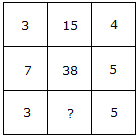# Verbal Reasoning - Character Puzzles - Discussion

### Discussion :: Character Puzzles - Character Puzzles 1 (Q.No.24)

24.

Which one will replace the question mark ?[A]. 15 [B]. 19 [C]. 20 [D]. 18

Explanation:

(3 x 4) + 3 = 15

and (7 x 5) + 3 = 38

Therefore (3 x 5) + 3 = 18.